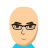首页   注册   登录cngithub 最近的时间轴更新# cngithub

•   ANREN
V2EX 第 12173 号会员，加入于 2011-09-28 22:57:05 +08:00
6469cngithub 创建的所有主题提问技术话题好玩工作信息交易信息城市相关
 iMac 升级OS X Mavericks 体验 macOS  •  cngithub  •  最后回复来自 wang2191195 3
 Markdown生态链整理  3    Markdown  •  cngithub  •  最后回复来自 hit9 15
 Markdown科技写作专业玩法 Markdown  •  cngithub  •  最后回复来自 yejian9237 4
 新款iMac能否直接当外接显示器使用？ iMac  •  cngithub  •  最后回复来自 alex99xu 2
 纪念华君 随想  •  cngithub  •  最后回复来自 iiduce 1
 为什么Markdown+R有较大概率成为科技写作主流？ Markdown  •  cngithub  •  最后回复来自 skywalker 23
 关于ios app排名的问题 问与答  •  cngithub  •  最后回复来自 reinhard 1
 如何避免思维进入死胡同 分享发现  •  cngithub  •  最后回复来自 hqcaptain 18
 如何学习科学：开放科学工具箱 分享创造  •  cngithub  •  最后回复来自 DXpro 6
 又被星光社抄袭个人博客文章了 自言自语  •  cngithub  •  最后回复来自 aveline 1
cngithub 最近回复了
 2013-11-04 13:30:24 +08:00
 2013-10-23 09:36:02 +08:00
 2013-08-13 12:42:49 +08:00 回复了 yt1988 创建的主题 › macOS › 迅雷 Mac 2.0的内测版来了
下了。很不错。界面精简得都不像迅雷了:D
 2013-07-26 19:58:54 +08:00
写过个类似APP。

如果是Ruby，大约不到千行代码解决。Python应该也差别不大。

选型Ruby，社区较流行的方案是：devise（解决一堆用户注册、授权等问题，移动端也解决）、角色管理则用Cancan等兼容Devise组合；API用grape。

Ruby部署与维护则非常方便，一般用vagrant+chef+capistrano实现一键分发与维护。

过些日子，我会放出我的维护项目：Railsbox，一键部署常见云主机。
 2013-07-25 14:24:14 +08:00 回复了 Livid 创建的主题 › V2EX › 从办公室到 V2EX server 的延迟 :-)
PING hk.v2ex.com (180.210.229.136): 56 data bytes
64 bytes from 180.210.229.136: icmp_seq=0 ttl=53 time=54.245 ms
64 bytes from 180.210.229.136: icmp_seq=1 ttl=53 time=56.728 ms
64 bytes from 180.210.229.136: icmp_seq=2 ttl=53 time=53.827 ms
64 bytes from 180.210.229.136: icmp_seq=3 ttl=53 time=54.170 ms
64 bytes from 180.210.229.136: icmp_seq=4 ttl=53 time=54.086 ms
64 bytes from 180.210.229.136: icmp_seq=5 ttl=53 time=53.407 ms
64 bytes from 180.210.229.136: icmp_seq=6 ttl=53 time=53.556 ms
64 bytes from 180.210.229.136: icmp_seq=7 ttl=53 time=55.578 ms
 2013-07-25 14:22:32 +08:00 回复了 Livid 创建的主题 › V2EX › 从办公室到 V2EX server 的延迟 :-)
不挂vpn，北京联通光纤网的ping结果。。。

PING www.v2ex.com (69.28.49.228): 56 data bytes
64 bytes from 69.28.49.228: icmp_seq=0 ttl=51 time=214.059 ms
64 bytes from 69.28.49.228: icmp_seq=1 ttl=51 time=215.635 ms
64 bytes from 69.28.49.228: icmp_seq=2 ttl=51 time=213.522 ms
64 bytes from 69.28.49.228: icmp_seq=3 ttl=51 time=213.632 ms
64 bytes from 69.28.49.228: icmp_seq=4 ttl=51 time=214.353 ms
64 bytes from 69.28.49.228: icmp_seq=5 ttl=51 time=214.172 ms
64 bytes from 69.28.49.228: icmp_seq=6 ttl=51 time=213.665 ms
64 bytes from 69.28.49.228: icmp_seq=7 ttl=51 time=213.713 ms
64 bytes from 69.28.49.228: icmp_seq=8 ttl=51 time=213.804 ms
 2013-07-25 14:21:05 +08:00 回复了 Livid 创建的主题 › V2EX › 从办公室到 V2EX server 的延迟 :-)
日常挂vpn（东京linode）ping结果：

PING www.v2ex.com (69.28.49.228): 56 data bytes

64 bytes from 69.28.49.228: icmp_seq=0 ttl=52 time=229.190 ms
64 bytes from 69.28.49.228: icmp_seq=1 ttl=52 time=229.242 ms
64 bytes from 69.28.49.228: icmp_seq=2 ttl=52 time=230.449 ms
64 bytes from 69.28.49.228: icmp_seq=3 ttl=52 time=228.774 ms
64 bytes from 69.28.49.228: icmp_seq=4 ttl=52 time=229.870 ms
64 bytes from 69.28.49.228: icmp_seq=5 ttl=52 time=228.812 ms
64 bytes from 69.28.49.228: icmp_seq=6 ttl=52 time=228.768 ms
64 bytes from 69.28.49.228: icmp_seq=7 ttl=52 time=229.230 ms
64 bytes from 69.28.49.228: icmp_seq=8 ttl=52 time=229.148 ms
64 bytes from 69.28.49.228: icmp_seq=9 ttl=52 time=228.627 ms
64 bytes from 69.28.49.228: icmp_seq=10 ttl=52 time=228.485 ms
64 bytes from 69.28.49.228: icmp_seq=11 ttl=52 time=230.934 ms
64 bytes from 69.28.49.228: icmp_seq=12 ttl=52 time=229.104 ms
64 bytes from 69.28.49.228: icmp_seq=13 ttl=52 time=228.778 ms
64 bytes from 69.28.49.228: icmp_seq=14 ttl=52 time=228.795 ms
64 bytes from 69.28.49.228: icmp_seq=15 ttl=52 time=229.359 ms
64 bytes from 69.28.49.228: icmp_seq=16 ttl=52 time=229.236 ms
64 bytes from 69.28.49.228: icmp_seq=17 ttl=52 time=229.381 ms
64 bytes from 69.28.49.228: icmp_seq=18 ttl=52 time=229.455 ms
64 bytes from 69.28.49.228: icmp_seq=19 ttl=52 time=229.379 ms
64 bytes from 69.28.49.228: icmp_seq=20 ttl=52 time=228.963 ms
64 bytes from 69.28.49.228: icmp_seq=21 ttl=52 time=229.266 ms
64 bytes from 69.28.49.228: icmp_seq=22 ttl=52 time=229.411 ms
64 bytes from 69.28.49.228: icmp_seq=23 ttl=52 time=228.730 ms
64 bytes from 69.28.49.228: icmp_seq=24 ttl=52 time=229.135 ms
64 bytes from 69.28.49.228: icmp_seq=25 ttl=52 time=228.634 ms
64 bytes from 69.28.49.228: icmp_seq=26 ttl=52 time=229.169 ms
64 bytes from 69.28.49.228: icmp_seq=27 ttl=52 time=228.739 ms
64 bytes from 69.28.49.228: icmp_seq=28 ttl=52 time=230.877 ms
64 bytes from 69.28.49.228: icmp_seq=29 ttl=52 time=228.691 ms
64 bytes from 69.28.49.228: icmp_seq=30 ttl=52 time=228.961 ms
64 bytes from 69.28.49.228: icmp_seq=31 ttl=52 time=229.066 ms
64 bytes from 69.28.49.228: icmp_seq=32 ttl=52 time=229.028 ms
64 bytes from 69.28.49.228: icmp_seq=33 ttl=52 time=229.052 ms
64 bytes from 69.28.49.228: icmp_seq=34 ttl=52 time=228.748 ms
64 bytes from 69.28.49.228: icmp_seq=35 ttl=52 time=229.031 ms
64 bytes from 69.28.49.228: icmp_seq=36 ttl=52 time=229.025 ms
64 bytes from 69.28.49.228: icmp_seq=37 ttl=52 time=228.752 ms
64 bytes from 69.28.49.228: icmp_seq=38 ttl=52 time=230.210 ms
64 bytes from 69.28.49.228: icmp_seq=39 ttl=52 time=228.536 ms
64 bytes from 69.28.49.228: icmp_seq=40 ttl=52 time=229.091 ms
64 bytes from 69.28.49.228: icmp_seq=41 ttl=52 time=228.698 ms
64 bytes from 69.28.49.228: icmp_seq=42 ttl=52 time=228.956 ms
64 bytes from 69.28.49.228: icmp_seq=43 ttl=52 time=228.798 ms
64 bytes from 69.28.49.228: icmp_seq=44 ttl=52 time=229.300 ms
64 bytes from 69.28.49.228: icmp_seq=45 ttl=52 time=229.285 ms
64 bytes from 69.28.49.228: icmp_seq=46 ttl=52 time=229.163 ms
64 bytes from 69.28.49.228: icmp_seq=47 ttl=52 time=228.888 ms
64 bytes from 69.28.49.228: icmp_seq=48 ttl=52 time=228.906 ms
64 bytes from 69.28.49.228: icmp_seq=49 ttl=52 time=228.847 ms
 2013-07-23 13:21:37 +08:00 回复了 turing 创建的主题 › Node.js › 使用命令行一键推送本地文件到 kindle
 2013-07-23 12:18:10 +08:00 回复了 Eyon 创建的主题 › MacBook Pro › 新 Air 上市了....
等了很久，送家属的。已下单。
关于   ·   FAQ   ·   API   ·   我们的愿景   ·   广告投放   ·   感谢   ·   实用小工具   ·   1070 人在线   最高记录 4385   ·创意工作者们的社区